# Polar To Rectangular

Polar To Rectangular

Rectangular coordinates, also known as Cartesian coordinates were first given by Rene Descartes in the 17th century. It is said Sir Isaac Newton was the one who developed 10 different coordinate systems, one among them being the polar coordinate system.

In this short lesson, you will be introduced to the method of converting polar coordinates to rectangular coordinates. There are simple formulas that can be used to convert polar coordinates to their respective rectangular coordinates. You will learn how to apply these formulas in problems to find the rectangular coordinates. Now, look at the figure below and try to identify the rectangular coordinates and the polar coordinates.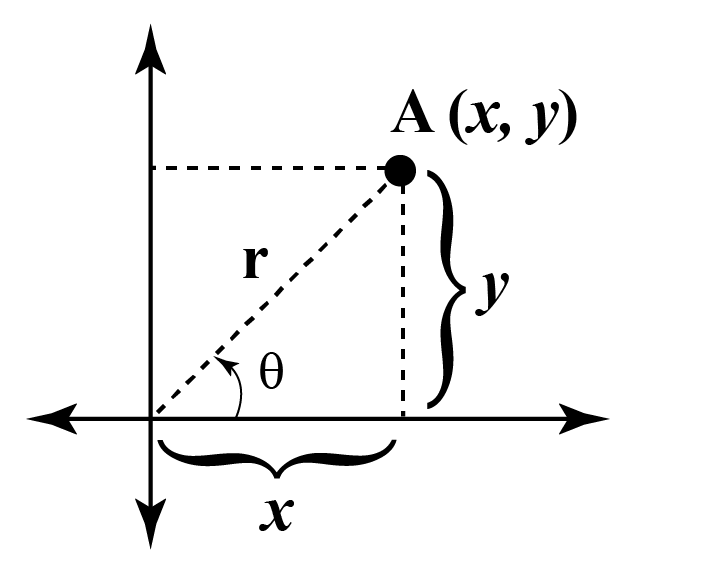## How to Convert Polar Coordinates to Rectangular Coordinates?

Polar coordinates are expressed as ($$r, \theta$$) while rectangular coordinates are expressed as ($$x, y$$).

Converting polar to rectangular coordinates means expressing the polar coordinates in the form of rectangular coordinates.

It is possible to convert polar to rectangular coordinates and vice versa.

## What Are the Formulas to Convert Polar Coordinates to Rectangular Coordinates?

Let's say for a two-dimensional system:

1. There is a point $$A$$ with coordinates ($$x, y$$).
2. The distance from the origin (0,0) to the point $$A$$ is given as $$r$$.
3. The line joining the origin to the point $$A$$ makes an angle $$\theta$$ with the positive $$x$$-axis.

The polar coordinates are given as ($$r, \theta$$) and rectangular coordinates are given as ($$x, y$$).The conversion formulas for polar to rectangular coordinates are given as:

 $$x = r\cos\theta$$ $$y = r\sin\theta$$Tips and Tricks
• The conversion formulas for rectangular to polar coordinates are given as, $$r = \sqrt{x^2 + y^2}$$ and $$\theta = \tan^{-1}(\frac{y}{x})$$.
• Take a look at the coordinate planes to know more.

### Examples

Example 1. The distance of a point $$B$$ from the origin is 4 units and the angle made with the positive $$x$$-axis is $$\frac{\pi}{3}$$.

Find the rectangular coordinates of point $$B$$.

Solution:

Distance of point B from the origin, $$r$$ = 4 units

Angle made with the positive $$x$$-axis, $$\theta$$ = $$\frac{\pi}{3}$$

The polar coordinates are ($$4, \frac{\pi}{3}$$).

We need to find the rectangular coordinates of $$B$$.

Let's say the rectangular coordinates of $$B$$ are given as ($$x, y$$).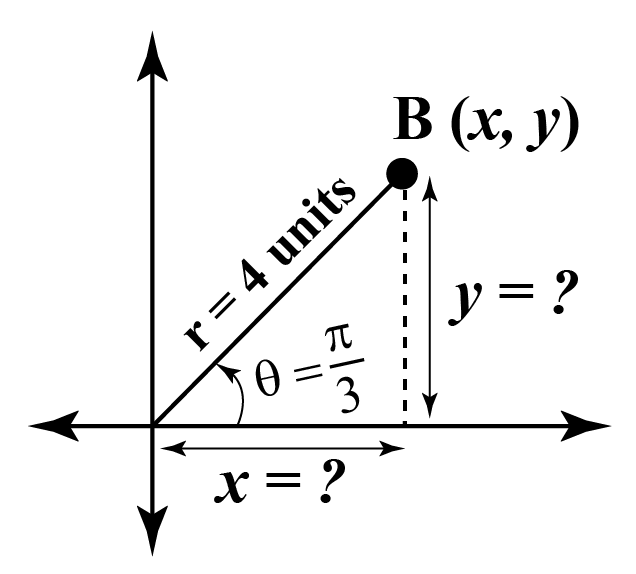Applying the polar to rectangular equations and substituting the trigonometric values for point $$B$$,

\begin{align}x &= r\cos\theta = 4 \times \cos\frac{\pi}{3} \\&= 4 \times \frac{1}{2} \\&= 2\end{align}

\begin{align}y &= r\sin\theta = 4 \times \sin\frac{\pi}{3} \\&= 4 \times \frac{\sqrt{3}}{2} \\&= 2\sqrt{3}\end{align}

Hence, the rectangular coordinates of $$B$$ are ($$2, 2\sqrt{3}$$).

Example 2. The distance of a point $$A$$ from the origin is 2 units and distance of a point $$B$$ from the origin is 5 units.

The angle made by the line $$OA$$ and $$OB$$ with the positive $$x$$-axis are $$\frac{\pi}{3}$$ and  $$\frac{\pi}{4}$$ respectively.

Find the rectangular coordinates of point $$A$$ and $$B$$.

Solution:

Distance of point $$A$$ from the origin, $$r_1 = 2$$ units

Angle made with the positive $$x$$-axis, $$\theta_1 = \frac{\pi}{3}$$

The polar coordinates are ($$2, \frac{\pi}{3}$$).

Distance of point B from the origin, $$r_2 = 5$$ units

Angle made with the positive $$x$$-axis, $$\theta_2 = \frac{\pi}{4}$$

The polar coordinates are ($$5, \frac{\pi}{4}$$).

We need to find the rectangular coordinates of $$A$$ and $$B$$.

Let's say the rectangular coordinates of $$A$$ are given as ($$x_1, y_1$$) and of $$B$$ are ($$x_2, y_2$$).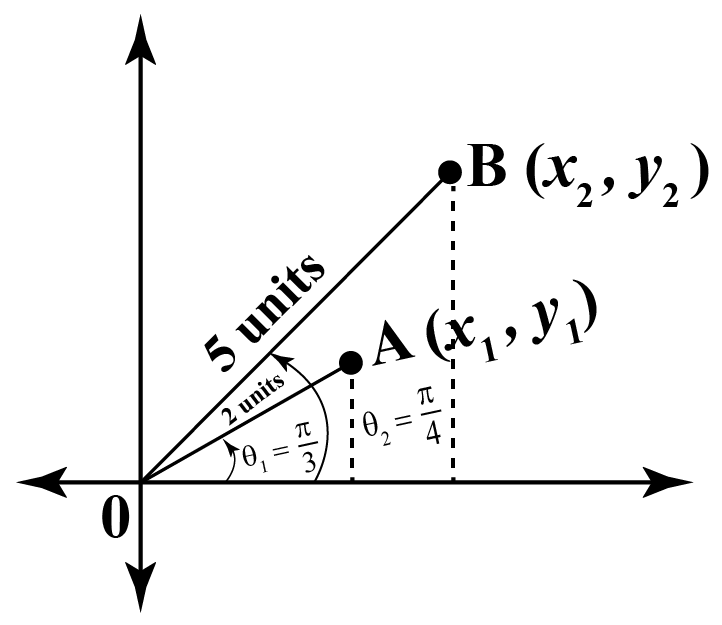Using the polar to rectangular equations and substituting the trigonometric values for point $$A$$ we get,

\begin{align}x &= r\cos\theta = 2 \times \cos\frac{\pi}{3} \\&= 2 \times \frac{1}{2} \\&= 1\end{align}

\begin{align}y &= r\sin\theta = 2 \times \sin\frac{\pi}{3} \\&= 2 \times \frac{\sqrt{3}}{2} \\&= \sqrt{3}\end{align}

Hence, the rectangular coordinates of $$A$$ are ($$1, \sqrt{3}$$).

Applying the polar to rectangular formulas and substituting the trigonometric values for point $$B$$ we get,

\begin{align}x &= r\cos\theta = 5 \times \cos\frac{\pi}{4} \\&= \frac{5}{\sqrt{2}}\end{align}

\begin{align}y &= r\sin\theta = 5 \times \sin\frac{\pi}{4} \\&= \frac{5}{\sqrt{2}}\end{align}

Hence, the rectangular coordinates of $$B$$ are ($$\frac{5}{\sqrt{2}}, \frac{5}{\sqrt{2}}$$).

## Method to Convert Polar to Rectangular Coordinates

We represent polar and rectangular coordinates using the following graph: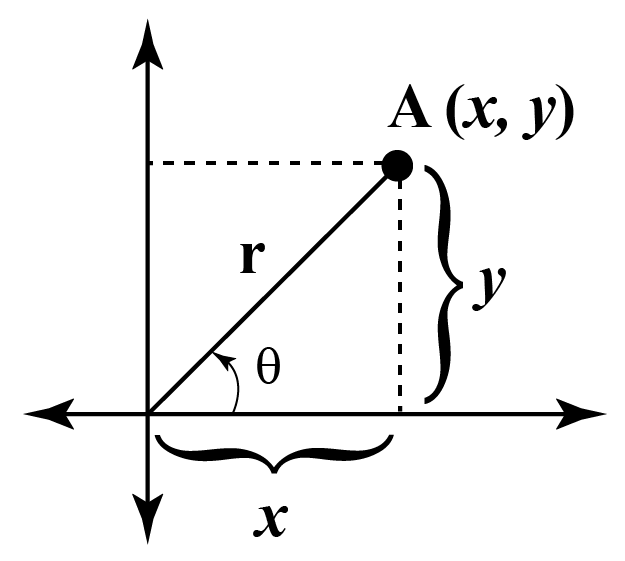We can observe that $$r$$ forms a right-angled triangle with $$x$$-axis.

As per the trigonometric ratios,

$$\cos\theta = \frac{x}{r}$$

$$\sin\theta = \frac{y}{r}$$

Both the above equations can be expressed in terms of $$x$$ and $$y$$ as,

$$x = r\cos\theta$$

$$y = r\sin\theta$$

The above derived equations are known as the polar to rectangular formulas.

### Examples

Example 1: The distance of point $$A$$ from the origin is 3 units and the distance of point $$B$$ from the origin is 4 units.

The angle made by the line $$OA$$ with the negative $$x$$-axis is $$\frac{\pi}{3}$$ and the angle made by the line $$OB$$ with the positive $$x$$-axis is $$\frac{\pi}{3}$$.

Find the rectangular coordinates of point $$A$$ and $$B$$.

Solution:

Distance of point A from the origin, $$r_1 = 3$$ units

Angle made with the negative $$x$$-axis, $$\theta_1 = \frac{\pi}{3}$$

Hence, the angle made with the positive $$x$$-axis is given as, $$\theta_1 = \pi - \frac{\pi}{3} = \frac{2\pi}{3}$$

The polar coordinates are ($$3, \frac{2\pi}{3}$$).

Distance of point B from the origin, $$r_2 = 4$$ units

Angle made with the positive $$x$$-axis, $$\theta_2 = \frac{\pi}{3}$$

The polar coordinates are ($$4, \frac{\pi}{3}$$).

We have to find the rectangular coordinates of $$A$$ and $$B$$.

Let's say the rectangular coordinates of $$A$$ are given as ($$x_1, y_1$$) and $$B$$ are given as ($$x_2, y_2$$).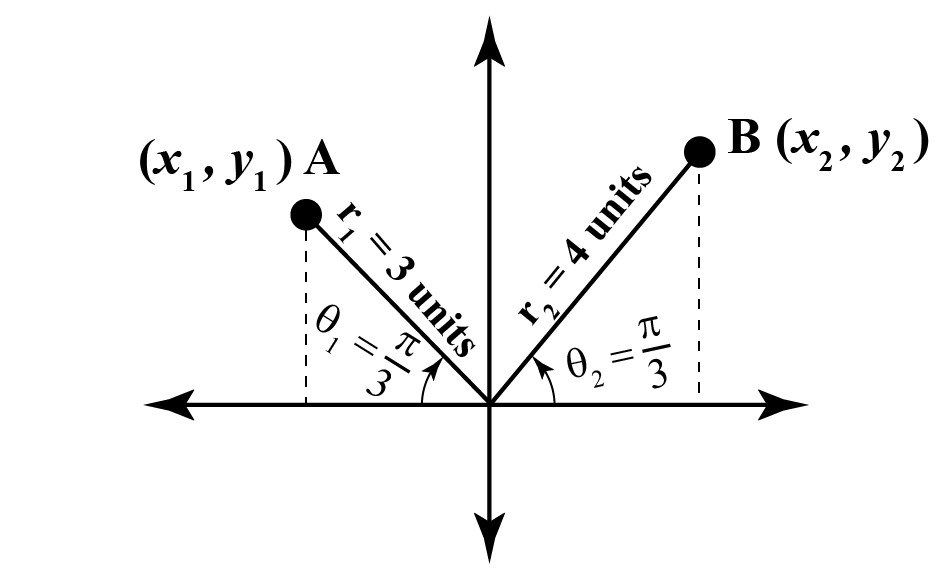Applying the polar to rectangular equations and substituting the trigonometric values for point $$A$$ we get,

\begin{align}x &= r\cos\theta = 3 \times \cos\frac{2\pi}{3} \\&= 3 \times \frac{-1}{2} \\&= \frac{-3}{2}\end{align}

\begin{align}y &= r\sin\theta = 3 \times \sin\frac{2\pi}{3} \\&= 3 \times \frac{\sqrt{3}}{2} \\&= \frac{3\sqrt{3}}{2}\end{align}

Hence, the rectangular coordinates of $$A$$ are ($$\frac{-3}{2}, \frac{3\sqrt{3}}{2}$$).

Applying the polar to rectangular formulas and substituting the trigonometric values for point $$B$$ we get,

\begin{align}x &= r\cos\theta = 4 \times \cos\frac{\pi}{3} \\&= 4 \times \frac{1}{2} \\&= 2\end{align}

\begin{align}y &= r\sin\theta = 4 \times \sin\frac{\pi}{3} \\&= 4 \times \frac{\sqrt{3}}{2} \\&= 2\sqrt{3}\end{align}

Hence, the rectangular coordinates of $$B$$ are ($$2, 2\sqrt{3}$$).

Example 2: Find the rectangular coordinates of a point lying on the $$y$$-axis with a distance $$r$$ from the origin.

Solution:

Point $$A$$ lies on the $$y$$-axis.

Hence, the angle between the point and the positive $$x$$-axis is $$\frac{\pi}{2}$$.

The distance of point $$A$$ from the origin is $$r$$.

The polar coordinates are ($$r, \frac{\pi}{2}$$).

We have to find the rectangular coordinates of $$A$$.

Let's say the rectangular coordinates of $$A$$ are given as ($$x, y$$).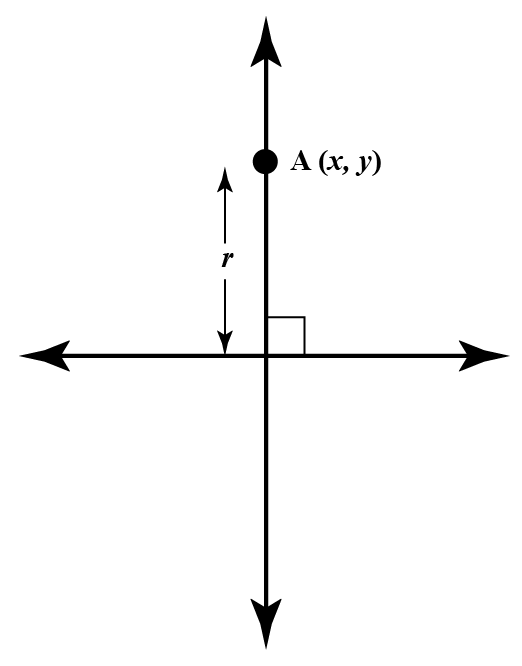Applying the polar to rectangular equations and substituting the trigonometric values for point $$A$$ we get,

\begin{align}x &= r\cos\theta = r \times \cos\frac{\pi}{2} \\&= r \times 0 \\&= 0\end{align}

\begin{align}y &= r\sin\theta = r \times \sin\frac{\pi}{2} \\&= r \times 1 \\&= r\end{align}

Hence, the rectangular coordinates of $$A$$ are ($$0, r$$) which also proves the reason every point on the $$y$$-axis only has the $$y$$ coordinate and no $$x$$ coordinate.

More Important Topics
More Important Topics